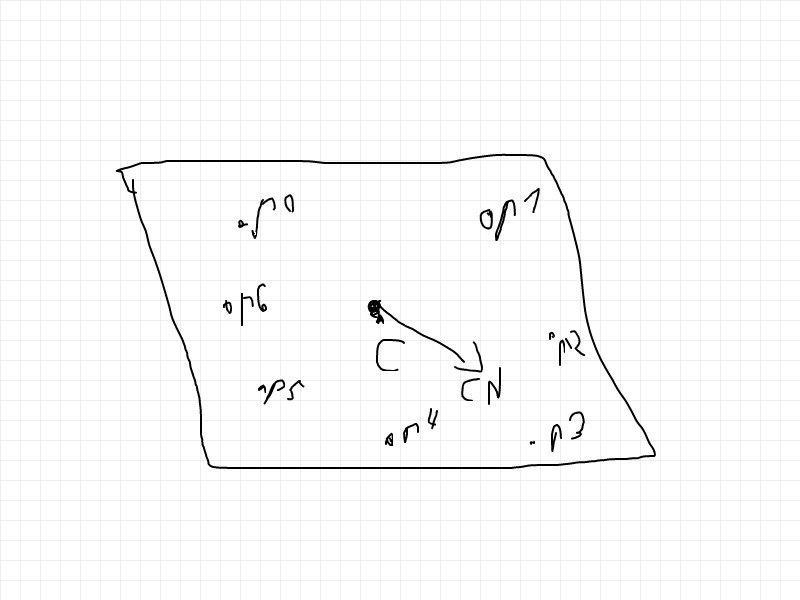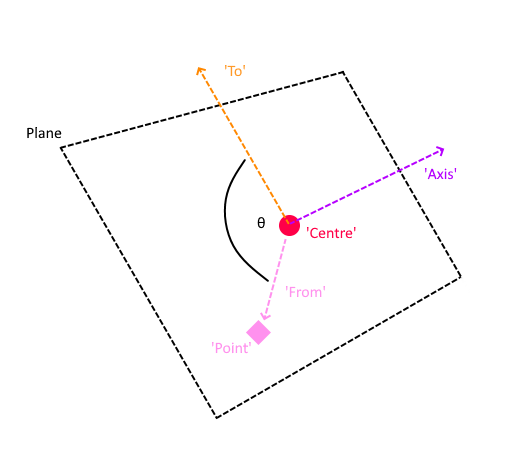# How to calculate 3d point angles on a plane in respect to centroid?

So I have a bunch of 3d points (Pn) that are more or less aligned on virtual plane.
Then from those points I calculate the centroid C (by average) and average normal vector CN (sorry it’s drawn very wrong).Now I want to calculate the angles of each Pn in respect to centroid and the centroid normal. Like P1 could be 45 degrees, P4 180, etc.

I think that this topic contains an answer to this problem: math - New to Lua - Get angle between two 3D Vectors - Stack Overflow.

``````-- Where a and b are 2 points as Vector3's
local angleInRadians = math.acos(a:Dot(b) / (a.Magnitude * b.Magnitude))
``````

Hmm… Can not relate my problem to that. I have to take into account 3 factors - point, centroid, centroid normal (for axis).

Well you don’t really need the centroid or point only the vector between them.

the point and centroid should be converted into a vector.

``````local a = point - centroid -- Points do not have angles so convert into a vector instead
local b = centroidNormal

local ans = math.acos( a:Dot(b) / (a.Magnitude * b.Magnitude) )
print(math.deg(ans))
``````

This will always give ~90 degrees no matter the point coordinates. Since the normal vector is “±” perpendicular to the plane. That’ wrong.

Well…in that case you shouldn’t really use normal then. Instead you have to make an “upwards” vector.

Is the centroid a CFrame or just a point in 3d space?

If it is a CFrame and if the plane is the same axis as two of the CFrame X,Y,Z axis you can simply select one of the CFrames Right,Up or Forward vectors that are parallel to the surface of the plane.

Why upwards? The plane can be in any orientation. Normal shows this orientation. And its not always one or another axis, it can be in between.

• Upwards I was refering to in 2d space.

If you look at heading you need the plane and you need an “upwards direction” to measure the angle of a point relative to another point.

You can see the “upwards” direction is North. This is something that you have to decide.

Direction should depend on the normal and not a constant. It can be like this for example! Up-right!

Slightly confused by what you what, but I’m guessing you want a signed angle?

``````local function sqrMagnitude(v)
return v.x * v.x + v.y * v.y + v.z * v.z
end

local function signedAngle(from, to, axis)
axis = axis or Vector3.FromNormalId(Enum.NormalId.Top)

local d = math.sqrt(sqrMagnitude(from) * sqrMagnitude(to))
if d > 1e-15 then
local angle = math.deg(math.acos(math.clamp(from:Dot(to) / d, -1, 1)))
local cross = from:Cross(to)
return angle * math.sign(axis.x * cross.x + axis.y * cross.y + axis.z * cross.z)
end

return 0
end

local centre = game.Workspace.Centre
local axis   = Vector3.FromNormalId(Enum.NormalId.Top)
local point  = game.Workspace.Point
point:GetPropertyChangedSignal('Position'):Connect(function ()
local direction = (centre.Position - point.Position).unit
local front     = Vector3.FromNormalId(Enum.NormalId.Front)
local angle     = signedAngle(direction, front, axis)

print(('Angle between centre & point is %s degrees'):format(('%.1f'):format(angle)))
end)
``````

For reference, this would measure angle such that:i.e. the points are rotated around the ‘axis’ normal, and the ‘to’ vector is the where we measure the angle. So if you’d need to provide a direction from the plane, or one perpendicular to the normal.